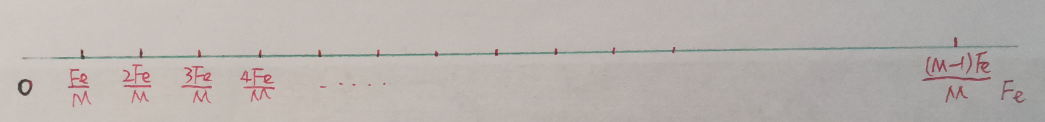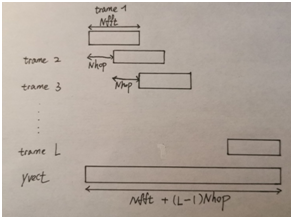# 短时傅里叶变换及其逆变换

## 一. 短时傅里叶变换与离散傅里叶变换

### 1.离散傅里叶变换

∀ k ∈ [ 0 , M − 1 ] , X [ k ] = ∑ n = 0 N − 1 x [ n ] e − 2 j π n f k F e = ∑ n = 0 N − 1 x [ n ] e − 2 j π n k F e M F e \\{\forall} k\in [0,M-1] ,X[k]=\sum^{N-1}_{n=0}x[n] e^{-\cfrac{2j\pi nf_k} {F_e}} = \sum^{N-1}_{n=0}x[n]e^{-\cfrac{2j\pi nk\frac{F_e}{M}}{Fe}}
= ∑ n = 0 N − 1 x [ n ] e − 2 j π k n M \qquad \qquad \qquad \qquad = \sum^{N-1}_{n=0}x[n]e^{-\cfrac{2j\pi kn}{M}}X[k]也可以理解为包含了M个复数值的向量。在离散傅里叶变换中，采样点的个数N(时域上的取样长度)，以及频域上的采样个数M都是可调整的，两个采样个数的选择对于DFT的解析度和精确度会有影响，这里就不过多展开。

### 2.短时傅里叶变换

X ( τ , f ) = ∫ R x ( t ) h ∗ ( t − τ ) e − 2 j π f t d t X(\tau,f)=\int_Rx(t)h^*(t-\tau)e^{-2j\pi ft}dt

# 二. 使用python 进行短时傅里叶变换

import numpy as np
import matplotlib.pyplot as plt
from scipy.io import wavfile
from IPython.display import display, Audio
from numpy import log10


def TFCT(trame, Fe, Nfft,fenetre,Nwin,Nhop):
L = round((len(trame) - len(fenetre))/Nhop)+1
M = Nfft
xmat = np.zeros((M,L))
print('xmat',xmat.shape)
print(Nwin+Nhop)
for j in range(L):
xmat[:,j] = np.fft.fft(trame[j*Nhop:Nwin+Nhop*j]*fenetre,Nfft)
x_temporel = np.linspace(0,(1/Fe)*len(trame),len(trame))
x_frequentiel = np.linspace(0, Fe,Nfft)

return xmat,x_temporel,x_frequentiel


trameFe : 初始的数字信号和它的采样频率
Nfft : 上文提到的离散傅里叶变换中，频域的采样个数M
fenetre : 短时傅里叶变换中使用的窗函数，在接下来的实现中我都使用了汉明窗np.hamming。
Nwin : 窗函数的长度(包含点的个数)
Nhop : 窗函数每次滑动的长度，一般规定为Nwin/2，窗函数长度的一半

# 三. 使用overlapp-add算法进行短时傅里叶变换的逆变换重构原信号

def ITFD(xmat,Fe,Nfft,Nwin,Nhop):
window = np.hamming(Nwin)
Te = 1/Fe
yvect = np.zeros(Nfft + (xmat.shape-1)*Nhop,dtype=complex)
t_vecteur = np.arange(0,Te*len(yvect),Te)
index = 0
K = 0
L = xmat.shape
yl = np.zeros(xmat.shape,dtype=complex)
for j in range(L):
yl[:,j] = np.fft.ifft(xmat[:,j])

# 平移和求和
for k in range(L):
yvect[Nhop*k:Nfft+Nhop*k] += yl[:,k]
# 标准化幅值
for n in range(Nwin-1):
K +=  window[n]
K /= Nhop
yvect /=K
return t_vecteur, yvect


## 1. 快速傅里叶逆变换

yl = np.zeros(xmat.shape,dtype=complex)
for j in range(L):
yl[:,j] = np.fft.ifft(xmat[:,j])


## 2. 对各列进行平移并叠加

 # 平移和求和
for k in range(L):
yvect[Nhop*k:Nfft+Nhop*k] += yl[:,k]## 3. 标准化

# 标准化幅值
for n in range(Nwin-1):
K +=  window[n]
K /= Nhop
yvect /=K
return t_vecteur, yvect


window[n] (w[n]) 是长度为Nwin的窗函数，在选取窗函数的时候，我们总满足规则   K = ∑ l = 1 L w [ n − ( l − 1 ) N h o p ] \ K=\sum^L_{l=1}w[n-(l-1)Nhop] K的值与n无关。在此基础上不难证明
K ≈ ∑ n = 0 N w i n − 1 w [ n ] / N h o p \ K \approx \sum^{Nwin-1}_{n=0}w[n] / Nhop11-15
06-18
04-29
10-22
12-124万+
04-03454
05-314万+
03-28545
08-0731万+
07-18
10-081022
11-283019
12-22306
01-126191
11-24484
04-081万+
05-08
04-25
©️2020 CSDN 皮肤主题: 点我我会动 设计师:白松林